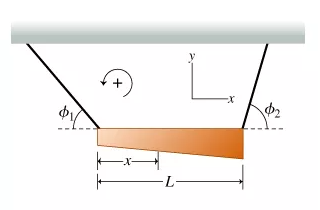# Problem: A nonuniform, horizontal bar of mass m is supported by two massless wires against gravity. The left wire makes an Φ1 with the horizontal, and the right wire makes an Φ2. The bar has length L.Find the position of the center of mass of the bar, x, measured from the bar's left end. Express the center of mass in terms of L, Φ1, and Φ2.

###### FREE Expert Solution

Let the tension on the left wire be TL and tension in the right wire be TR

Balancing the forces in the horizontal direction gives:

$\begin{array}{rcl}\begin{array}{rc}{\mathbf{T}}_{\mathbf{R}}\mathbf{c}\mathbf{o}\mathbf{s}{\mathbf{\varphi }}_{\mathbf{2}}& \mathbf{-}{\mathbf{T}}_{\mathbf{L}}\mathbf{c}\mathbf{o}\mathbf{s}{\mathbf{\varphi }}_{\mathbf{1}}\end{array}& \mathbf{=}& \mathbf{0}\\ {\mathbf{T}}_{\mathbf{R}}& \mathbf{=}& \frac{{\mathbf{T}}_{\mathbf{L}}\mathbf{c}\mathbf{o}\mathbf{s}{\mathbf{\varphi }}_{\mathbf{1}}}{\mathbf{c}\mathbf{o}\mathbf{s}{\mathbf{\varphi }}_{\mathbf{2}}}\end{array}$

88% (348 ratings)###### Problem Details

A nonuniform, horizontal bar of mass m is supported by two massless wires against gravity. The left wire makes an Φwith the horizontal, and the right wire makes an Φ2. The bar has length L.Find the position of the center of mass of the bar, x, measured from the bar's left end. Express the center of mass in terms of L, Φ1, and Φ2.